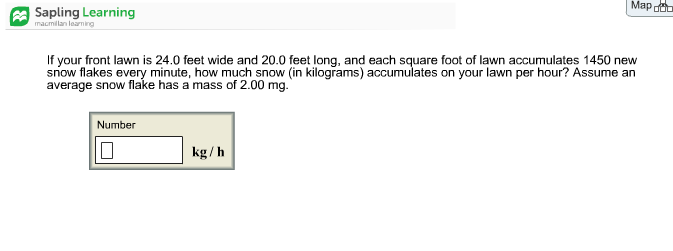# Question & Answer: Map Sapling Learning macmilan learning If your front lawn is 24.0 feet wide and 20.0 feet…..Map Sapling Learning macmilan learning If your front lawn is 24.0 feet wide and 20.0 feet long, and each square foot of lawn accumulates 1450 new snow flakes every minute, how much snow (in kilograms) accumulates on your lawn per hour? Assume an average snow flake has a mass of 2.00 mg. Number kg/h

area of front lawn = 24*20 = 480 ft2

Don't use plagiarized sources. Get Your Custom Essay on
Question & Answer: Map Sapling Learning macmilan learning If your front lawn is 24.0 feet wide and 20.0 feet…..
GET AN ESSAY WRITTEN FOR YOU FROM AS LOW AS \$13/PAGE

in each square feet, 1450 new snow flakes every minute

So, snow flake rate = 480*1450 = 696000/min = 696000*60/hour = 4.176*107/hour

each snow flake weight = 2 mg = 2*10-3 g = 2*10-6 kg

So snow flake rate = (4.176*107)*(2*10-6 kg)/hour = 83.52 kg/hour

in one hour, 83.52 kg snow flake accumulated